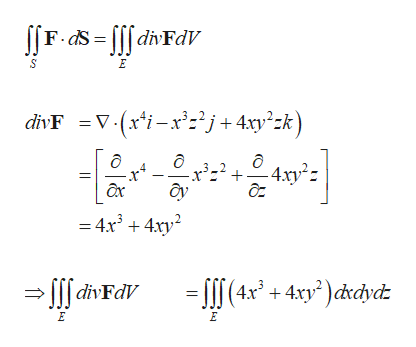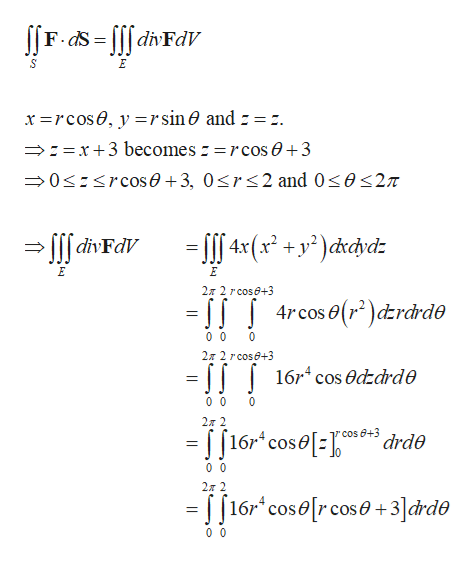# Use the Divergence Theorem to calculate the surface integral   SF · dS; that is, calculate the flux of F across S.F(x, y, z) = x4i − x3z2j + 4xy2zk,S is the surface of the solid bounded by the cylinder x2 + y2 = 4 and the planes z = x + 3 and z = 0.

Question
60 views

Use the Divergence Theorem to calculate the surface integral

 S
F · dS;

that is, calculate the flux of F across S.

F(x, y, z) = x4i − x3z2j + 4xy2zk,

S is the surface of the solid bounded by the cylinder
x2 + y2 = 4
and the planes
z = x + 3 and z = 0.
check_circle

Step 1

Apply the Divergence Theorem as follows.help_outlineImage TranscriptioncloseF.dS = [[[ divFdV divF =V (x*i-x°z*j+ 4xy°zk) -x²=² + ôy - 4.xy²z = 4x + 4xy? = [[[(4x* + 4xy° )c&rdycz → > ||| divFdV fullscreen
Step 2

Use the cylindrical coordinates as ...help_outlineImage TranscriptioncloseJ|F-as = [[f divFdV x =rcos0, y =rsin0 and - =:. →:=x+3 becomes :=rcos e +3 →0<:

### Want to see the full answer?

See Solution

#### Want to see this answer and more?

Solutions are written by subject experts who are available 24/7. Questions are typically answered within 1 hour.*

See Solution
*Response times may vary by subject and question.
Tagged in

### Math